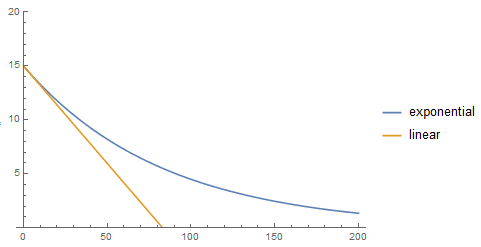# Damped harmonic oscillation of a swingboat

• orangephysik

#### orangephysik

Homework Statement
A swingboat with mass m = 130 kg is approximately taken to be a mathematical pendulum with a suspension length l = 3.5 m.
The swingboat undergoes a damped harmonic oscillation after the motor is turned off (at t = 0) with the form Φ(t) = Φ_0 * exp(-γt)*cos(ωt) with a starting amplitude Φ(t=0) = 15° and a frequency of f = 0.27 Hz. The amplitude is reduced to 12° after 5 oscillation periods.

a) Calculate the damping coefficient γ
b) How long does it take until the amplitude reaches 2° ?
Relevant Equations
T = 1/f
Hi,

so of course Φ0 = 15° and after solving after solving Φ(t=5*T = 5/f) I found γ = 0.012
I need help with b).
If I do 2° = 15° * exp(-0.012t)*cos(2πf*t), I'm not able to find t so I did something else by assuming that the amplitude decreases at a constant rate:

After 5*T = 5*1/f = 18.52 s, the amplitude decreases by 3°.
So I have 18.52 s --> ΔΦ = 3°.
Which means after 1 s the amplitude decreases by 3°/18.52 = 0.162°

15° - 0.162°*x = 2° ⇔ x = 80.25
Which means it would take 80.25 s. But if I plug in t = 80.25 s into Φ(t) = Φ_0 * exp(-γt)*cos(ωt) I don't get 2° so my method was wrong.

I would like to ask why my method is wrong and how I could solve this problem. How could I solve the equation 2° = 15° * exp(-0.012t)*cos(2πf*t)? I thought I could use Euler's formula for cos(2πf*t) so that I could write the right hand side of the equation as a single e to the power of something, but it gets me nowhere.

Take the amplitude of the oscillation to be given by Φ_0 * exp(-γt). Find the time when this equals 2o.

Take the amplitude of the oscillation to be given by Φ_0 * exp(-γt). Find the time when this equals 2o.
I got t = 167.9 s.
So I guess by omitting the cos term), we only consider the part when the pendulum swings back to its neutral position for the first time (I can see now why my assumption was false). But t =167.9 s doesn't seem right, since it only takes 1/f = 1/(0,27 Hz) = 3.70 s for one Period.
Perhaps I calculated γ wrong?
I did Φ(t=5*T = 5/f) = 15° * exp((-5/f)*γ)*cos(2πf*5/f)
⇔ 12° = 15°*exp((-5/f)*γ)
⇔ ln(12°/15°) = (-5/f)*γ
⇔ γ = 0.0120498
seems correctThe damping is exponential, not linear.I got t = 167.9 s.
So I guess by omitting the cos term), we only consider the part when the pendulum swings back to its neutral position for the first time (I can see now why my assumption was false). But t =167.9 s doesn't seem right, since it only takes 1/f = 1/(0,27 Hz) = 3.70 s for one Period.
Perhaps I calculated γ wrong?
I did Φ(t=5*T = 5/f) = 15° * exp((-5/f)*γ)*cos(2πf*5/f)
⇔ 12° = 15°*exp((-5/f)*γ)
⇔ ln(12°/15°) = (-5/f)*γ
⇔ γ = 0.0120498
seems correctI get 167.0, by an easier method.
5 swings attenuates it by the ratio 4/5.
5n swings attenuates it by the ratio (4/5)n=2/15.
##n=\frac{\ln(2/15)}{\ln(4/5)}##.

•nasu and TSny
I get 167.0, by an easier method.
5 swings attenuates it by the ratio 4/5.
5n swings attenuates it by the ratio (4/5)n=2/15.
##n=\frac{\ln(2/15)}{\ln(4/5)}##.
I can't comprehend the logic behind this, could you explain how you did that?

And is 167.0 s the correct answer? :) (Well technically 167.2 s if I plug in the exact values, and I get the same value too if I use the exact value of γ when solving 2=Φ_0 * exp(-γt) ).

I can't comprehend the logic behind this, could you explain how you did that?
it is like radioactive decay law. You know, half life and such.